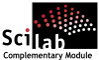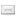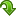Scilab Home Page | Wiki | Bug Tracker | Forge | Mailing List Archives | Scilab Online Help | File Exchange
ATOMS : Metanet details
Please login or create an account

# Metanet

Graph and Network toolbox
(17167 downloads for this version - 40463 downloads for all versions)Details
Version
0.6.2
Authors
Claude Gomez
Serge Steer
Maurice Goursat
Allan Cornet
Allan Simon
Bruno Jofret
Michael Baudin
Clément David
Owner Organization
Scilab Enterprises, DIGITEO, INRIA
Maintainers
Antoine Elias
Pierre Marechal
Michael Baudin
Clement David
Category
License
Creation Date
October 10, 2014
Source created on
Scilab 5.5.x
Binaries available on
Scilab 5.5.x:Linux 32-bitWindows 32-bitWindows 64-bitLinux 64-bit
Install command
`--> atomsInstall("metanet")`
Report a bugDescription
```            Metanet is a toolbox of Scilab for graphs and networks computations.
A number of algorithms solving classical graph problems and minimal cost flow
network are provided.

Features
--------

The following is a list of functions in this module.

* add_edge : adds an edge or an arc between two nodes
* add_edge_data : associates new data fields to the edges data structure of a
graph
* add_node : adds disconnected nodes to a graph
* add_node_data : associates new data fields to the nodes data structure of a
graph
* adj_lists : computes adjacency lists
* arc_graph : graph with nodes corresponding to arcs
* arc_number : number of arcs of a graph
* articul : finds one or more articulation points
* bandwr : bandwidth reduction for a sparse matrix
* best_match : maximum matching of a graph
* chain_struct : chained structure from adjacency lists of a graph
* check_graph : checks a Scilab graph data structure
* circuit : finds a circuit or the rank function in a directed graph
* con_nodes : set of nodes of a connected component
* connex : connected components
* contract_edge : contracts edges between two nodes
* convex_hull : convex hull of a set of points in the plane
* cycle_basis : basis of cycle of a simple undirected graph
* delete_arcs : deletes all the arcs or edges between a set of nodes
* delete_edges : deletes all the arcs or edges between a set of nodes
* delete_nodes : deletes nodes
* edge_number : number of edges of a graph
* edgedatafields : returns the vector of edge data fields names
* edges_data_structure : description of the data structure representing the
edges of a graph
* edit_graph : graph and network graphical editor
* edit_graph_menus : edit_graph menus description
* egraphic_data_structure : data structure representing the graphic properties
used for edges graphical display
* find_path : finds a path between two nodes
* gen_net : interactive or random generation of a network
* girth : girth of a directed graph
* glist : Scilab-4.x graph list creation
* graph-list : description of graph list (obsolete)
* graph_2_mat : node-arc or node-node incidence matrix of a graph
* graph_center : center of a graph
* graph_complement : complement of a graph
* graph_data_structure : description of the main graph data structure
* graph_diameter : diameter of a graph
* graph_power : kth power of a directed 1-graph
* graph_simp : converts a graph to a simple undirected graph
* graph_sum : sum of two graphs
* graph_union : union of two graphs
* hamilton : hamiltonian circuit of a graph
* hilite_edges : highlights a set of edges : unhighlights a set of edges
* hilite_nodes : highlights a set of nodes : unhighlights a set of nodes
* index_from_tail_head : Computes the index of edges given by (tail,head)
pairs
* is_connex : connectivity test
* knapsack : solves a 0-1 multiple knapsack problem
* line_graph : graph with nodes corresponding to edges
* load_graph : loads a graph from a file
* make_graph : makes a graph list
* mat_2_graph : graph from node-arc or node-node incidence matrix
* max_cap_path : maximum capacity path
* max_clique : maximum clique of a graph
* max_flow : maximum flow between two nodes
* mesh2d : triangulation of n points in the plane
* metanet_module_path : Returns the path of the metanet module
* min_lcost_cflow : minimum linear cost constrained flow
* min_lcost_flow1 : minimum linear cost flow
* min_lcost_flow2 : minimum linear cost flow
* min_qcost_flow : minimum quadratic cost flow
* min_weight_tree : minimum weight spanning tree
* neighbors : nodes connected to a node
* netclose : closes an edit_graph window
* netwindow : selects the current edit_graph window
* netwindows : gets the numbers of edit_graph windows
* ngraphic_data_structure : data structure representing the graphic properties
used for nodes graphical display
* node_number : number of nodes of a graph
* nodedatafields : returns the vector of node data fields names
* nodes_2_path : path from a set of nodes
* nodes_data_structure : description of the data structure representing the
nodes of a graph
* nodes_degrees : degrees of the nodes of a graph
* path_2_nodes : set of nodes from a path
* perfect_match : min-cost perfect matching
* pipe_network : solves the pipe network problem
* plot_graph : general plot of a graph (obsolete)
* predecessors : tail nodes of incoming arcs of a node
* qassign : solves a quadratic assignment problem
* salesman : solves the travelling salesman problem
* save_graph : saves a graph in a file
* set_nodes_id : displays labels near selected nodes in a graph display.
* shortest_path : shortest path
* show_arcs : highlights a set of arcs
* show_edges : highlights a set of edges
* show_graph : displays a graph
* show_nodes : highlights a set of nodes
* split_edge : splits an edge by inserting a node
* strong_con_nodes : set of nodes of a strong connected component
* strong_connex : strong connected components
* subgraph : subgraph of a graph
* successors : head nodes of outgoing arcs of a node
* supernode : replaces a group of nodes with a single node
* trans_closure : transitive closure
* update_graph : converts an old graph data structure to the current one.

Bibliography
------------

* "METANET : a system for network problems study", Claude Gomez, Maurice
Goursat, 1990
* "Metanet User's Guide and Tutorial", Claude Gomez, Maurice Goursat, 1998

Authors
-------

* Copyright (c) INRIA - Serge Steer
* Copyright (c) INRIA - Claude Gomez
* Copyright (c) INRIA - Maurice Goursat
* Copyright (c) 2008 - DIGITEO
* Copyright (c) 2010 - DIGITEO - Sylvestre Ledru
* Copyright (c) 2010 - DIGITEO - Allan Cornet
* Copyright (c) 2010 - DIGITEO - Allan Simon
* Copyright (c) 2010 - DIGITEO - Bruno Jofret
* Copyright (c) 2010 - DIGITEO - Michael Baudin
* Copyright (c) 2012 - Scilab Enterprises - Clement David
* Copyright (c) 2014 - Scilab Enterprises - Clement David

```Files (5)Source code archive
```
```Linux 32-bit binary for Scilab 5.5.x
```Linux 32-bit
Automatically generated by the ATOMS compilation chain

```Windows 32-bit binary for Scilab 5.5.x
```Windows 32-bit
Automatically generated by the ATOMS compilation chain

```Windows 64-bit binary for Scilab 5.5.x
```Windows 64-bit
Automatically generated by the ATOMS compilation chain

```Linux 64-bit binary for Scilab 5.5.x
```Linux 64-bit
Automatically generated by the ATOMS compilation chain

```News (0)Comments (1)Comment from Davide Cibecchini -- May 1, 2017, 03:32:25 PM
```

Really thanks for this.

I would like to know when will be released the Scilab 6.0 version.

I tried to fix the errors coming out on load, but I could only remove those on macros and
.start file due to the language changes and binaries update...

thank you```Leave a comment
You must register and log in before leaving a comment.Le compte avec l'identifiant 2114 n'existe pas